# NCERT Solutions For Class 6 Maths Decimals Exercise 8.5

NCERT Solutions For Class 6 Maths Decimals Exercise 8.5

### NCERT Solutions For Class 6 Maths Decimals Exercise 8.5

NCERT Solutions for Class 6 Maths Chapter 8 Decimals Ex 8.5

Exercise 8.5

Ex 8.5 Class 6 Maths Question 1.
Find the sum in each of the following:
(a) 0.007 + 8.5 + 30.08
(b) 15 + 0.632 + 13.8
(c) 27.076 + 0.55 + 0.004
(d) 25.65 + 9.005 + 3.7
(e) 0.75 + 10.425 + 2
(f) 280.69 + 25.2 + 38
Solution:
(a) 0.007 + 8.5 + 30.08
= 0.007 + 8.500 + 30.080 (making like decimals)
= 38.587(b) 15 + 0.632 + 13.8
= 15.000 + 0.632 + 13.800 (making like decimals)
= 29.432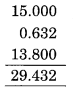(c) 27.076 + 0.55 + 0.004
= 27.076 + 0.550 + 0.004 (making like decimals)
= 27.630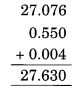(d) 25.65 + 9.005 + 3.7
= 25.650 + 9.005 + 3.700 (making like decimals)
= 38.355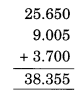(e) 0.75 + 10.425 + 2
= 0.750 + 10.425 + 2.000 (making like decimals)
= 13.175(f) 280.69 + 25.2 + 38
= 280.69 + 25.20 + 38.00 (making like decimals)
= 343.89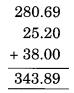Ex 8.5 Class 6 Maths Question 2.
Rashid spent ₹35.75 for Maths book and ₹32.60 for Science book. Find the total amount spent by Rashid.
Solution:
Money spent by Rashid for Maths book = ₹35.75
Money spent by Rashid for Science book = ₹32.60
∴ Total money spent by Rashid on both books = ₹35.75 + ₹32.60 = ₹68.35Ex 8.5 Class 6 Maths Question 3.
Radhika’s mother gave her ₹10.50 and her father gave her ₹15.80, find the total amount given to Radhika by her parents.
Solution:
Money given by Radhika’s mother = ₹10.50
Money given by her father = ₹15.80
∴ Total money given to her by her parents
= ₹10.50 + ₹15.80 = ₹26.30Ex 8.5 Class 6 Maths Question 4.
Nasreen bought 3 m 20 cm cloth for her shirt and 2 m 5 cm cloth for her trouser. Find the total length of cloth bought by her.
Solution:
Length of cloth bought by Nasreen for her shirt = 3 m 20 cm = 3.20 m
Length of cloth brought by her for her trouser = 2 m 5 cm = 2.05 m
Total length of cloth bought by her = 3.20 m + 2.05 m = 5.25 mEx 8.5 Class 6 Maths Question 5.
Naresh walked 2 km 35 m in the morning and 1 km 7 m in the evening. How much distance did he walk in all?
Solution:Ex 8.5 Class 6 Maths Question 6.
Sunita travelled 15 km 268 m by bus, 7 km 7 m by car and 500 m on foot in order to reach her school. How far is her school from her residence?
Solution: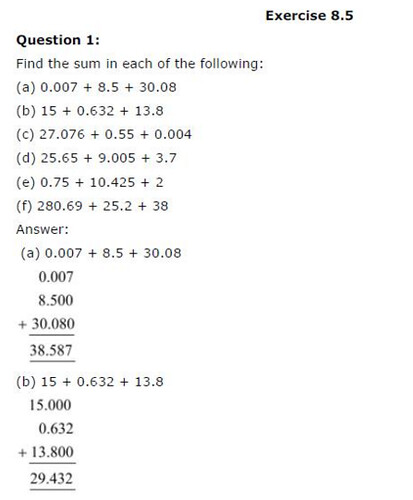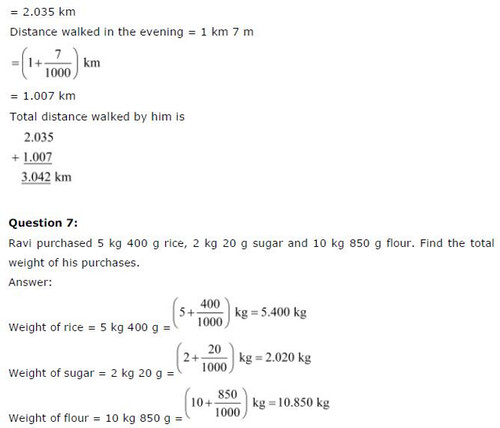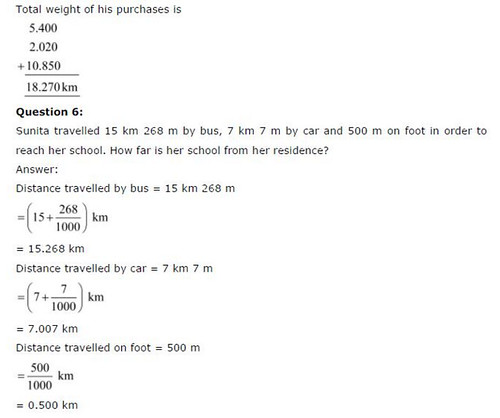## SabDekho

The Complete Educational Website